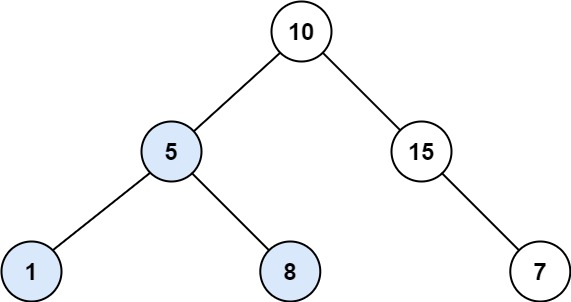### LeetCode• ㊗️
• 大家
• offer
• 多多！

## Problem

Given the root of a binary tree, find the largest subtree, which is also a Binary Search Tree (BST), where the largest means subtree has the largest number of nodes.

A Binary Search Tree (BST) is a tree in which all the nodes follow the below-mentioned properties:

The left subtree values are less than the value of their parent (root) node’s value. The right subtree values are greater than the value of their parent (root) node’s value. Note: A subtree must include all of its descendants.

Follow up: Can you figure out ways to solve it with O(n) time complexity?

Example 1:Input: root = [10,5,15,1,8,null,7]
Output: 3
Explanation: The Largest BST Subtree in this case is the highlighted one. The return value is the subtree's size, which is 3.


Example 2:

Input: root = [4,2,7,2,3,5,null,2,null,null,null,null,null,1]
Output: 2


## Code

class Solution {
class SearchNode{
int size;
int lower;
int upper;

SearchNode(int size, int lower, int upper){
// 表示子树的大小,如果是-1表示子树不是BST
this.size = size;
this.lower = lower;
this.upper = upper;
}
}

private SearchNode helper(TreeNode root){
if(root == null){
return new SearchNode(0, Integer.MAX_VALUE, Integer.MIN_VALUE);
}

SearchNode left = helper(root.left);
SearchNode right = helper(root.right);
// 遍历到当前的节点,必须要求这个点小于右子树的最小值, 大于左子树的最大值
if(left.size == -1 || right.size == -1 || root.val <= left.upper || root.val >= right.lower){
return new SearchNode(-1, 0, 0);
}

int size = left.size + right.size + 1;
res = Math.max(res, size);
return new SearchNode(size, Math.min(left.lower, root.val), Math.max(right.upper, root.val));
}

int res = 0;
public int largestBSTSubtree(TreeNode root) {
if(root == null) return 0;
helper(root);
return res;
}
}